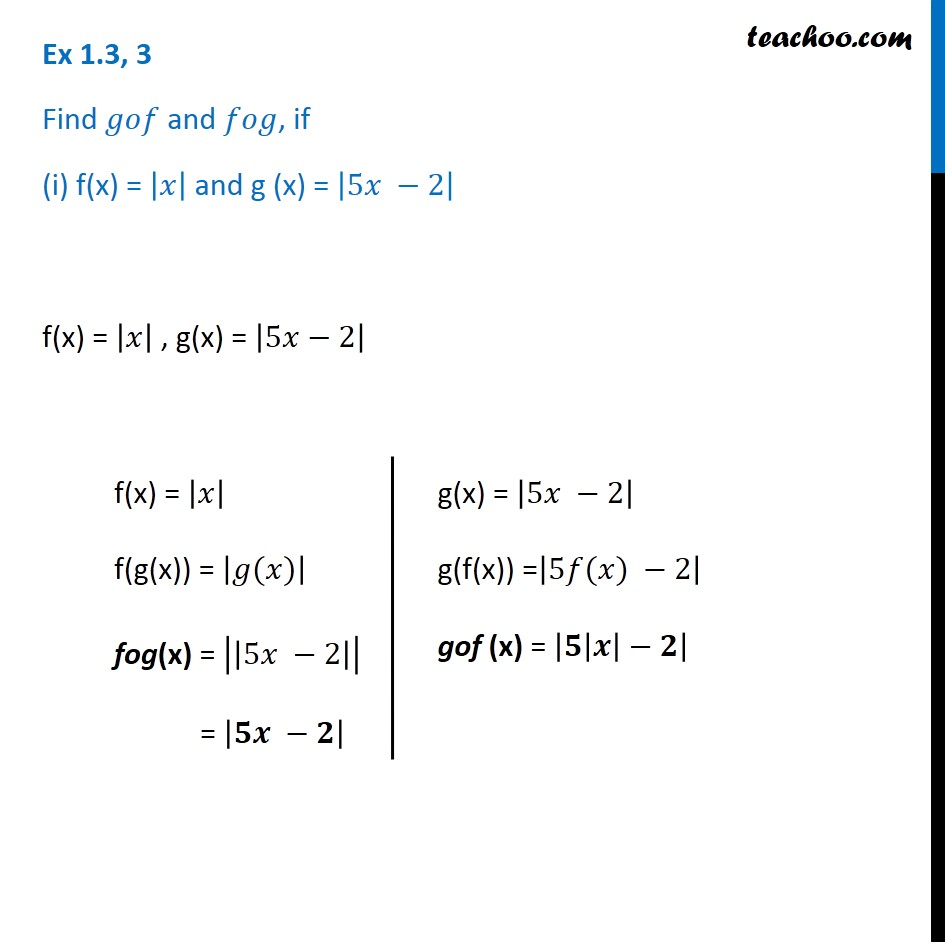Composite functions

Chapter 1 Class 12 Relation and Functions
Concept wiseIntroducing your new favourite teacher - Teachoo Black, at only ₹83 per month

### Transcript

Ex 1.3, 3 Find 𝑔𝑜𝑓 and 𝑓𝑜𝑔, if (i) f(x) = |𝑥| and g (x) = |5𝑥 −2| f(x) = |𝑥| , g(x) = |5𝑥−2| f(x) = |𝑥| f(g(x)) = |𝑔(𝑥)| fog(x) = |(|5𝑥 −2|)| = |𝟓𝒙 −𝟐| g(x) = |5𝑥 −2| g(f(x)) =|5𝑓(𝑥) −2| gof (x) = |(𝟓|𝒙|−𝟐)|